Courses

# Test: Nature Of Roots

## 25 Questions MCQ Test Mathematics (Maths) Class 10 | Test: Nature Of Roots

Description
This mock test of Test: Nature Of Roots for Class 10 helps you for every Class 10 entrance exam. This contains 25 Multiple Choice Questions for Class 10 Test: Nature Of Roots (mcq) to study with solutions a complete question bank. The solved questions answers in this Test: Nature Of Roots quiz give you a good mix of easy questions and tough questions. Class 10 students definitely take this Test: Nature Of Roots exercise for a better result in the exam. You can find other Test: Nature Of Roots extra questions, long questions & short questions for Class 10 on EduRev as well by searching above.
QUESTION: 1

Solution:
QUESTION: 2

### The real roots of a quadratic equation ax2 + bx + c = 0 are given by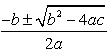Solution:

When b- 4ac=0 then we have the root as -b/2a.
When  b2-4ac < 0,then the root is a complex root ,since we have a negative number ,for which real square root is not possible.
When b- 4ac > 0 then we have a square root of it and hence we have real roots. So the correct answer is b- 4ac > 0

QUESTION: 3

### The solutions of the quadratic equation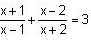are​

Solution: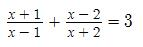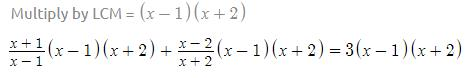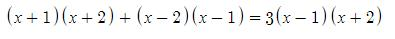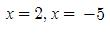QUESTION: 4

The value of b2 - 4ac  for equation 3x2 - 7x - 2 = 0 is

Solution:
QUESTION: 5

Which of the following is not a quadratic equation ?

Solution:
QUESTION: 6

For what value(s) of k will the equation kx2-5x+k = 0 have a repeated root?​

Solution:
QUESTION: 7

A real number is said to be a root of ax2+bx+c = 0

Solution:
QUESTION: 8

-3 is a root of the quadratic equation 2x2 +px – 15 = 0. For what value of q, the equation p(x2 + x ) + q = 0 has equal roots?​

Solution:
QUESTION: 9

The roots of x2 – 8x + 12 = 0, are

Solution:
QUESTION: 10

If 1/2 is a root of the equation x2+kx – (5/4) = 0, then the other root of the quadratic equation is​

Solution:
QUESTION: 11

The sum of the ages of two friends is 20 years. Four years ago, the product of their ages in years was 48. Find their present ages

Solution:
QUESTION: 12

The nature of the roots of following quadratic equation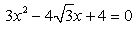Solution:
QUESTION: 13

If the equation px2 – 6x – 2 = 0 has real roots then, ________

Solution:
QUESTION: 14

The positive value of k, for which both equations: x2 + kx + 64 = 0 and x2 – 8x + k = 0 have real roots is_______

Solution:
QUESTION: 15

If one root of a Quadratic equation is m +, then the other root is​

Solution:

In a quadratic equation with rational coefficients has an irrational root  α + √β, then it has a conjugate root α - √β.
So if the root is m+ √n the other root will be m- √n

QUESTION: 16

If the value of the Discriminant function of a quadratic equation is D = 27, then its roots are

Solution:
QUESTION: 17

Comment on the nature of the roots of the equation 7x - 3x2 - 2 = 0​

Solution:
QUESTION: 18

The roots of the equation x2 – 3x – m (m + 3) = 0, where m is a constant, are​

Solution:

X2 - 3x - m(m+3) = 0

=> x2 + mx - (m+3)x- m(m+3) = 0

=> x(x+m) - (m+3)(x+m) = 0

=> (x+m) (x-m-3) = 0

x = - m and m+3

Roots are - m and m+3

QUESTION: 19

If the equation 9x2 – 6x + c = 0 has equal roots then, the value of c is

Solution:
QUESTION: 20

If -3 is a root of both the Quadratic equations 2×2 + px – 15 = 0, and p(x2 + x) + q = 0, then the value of q if the equation containing it has equal roots is

Solution:
QUESTION: 21

If a,b,c are real and b2-4ac >0 then roots of equation are​

Solution:
QUESTION: 22

The quadratic equation whose roots are real and equal is:​

Solution:
QUESTION: 23

Which of the following equations has two distinct real roots?​

Solution:
QUESTION: 24

If the equation 2x2 + 2(c - 20) x + (c - 20) = 0 has equal roots then, the value of c is

Solution:
QUESTION: 25

Which of the following is a solution of the quadratic equation x2 - b2 = a (2x - a)

Solution: